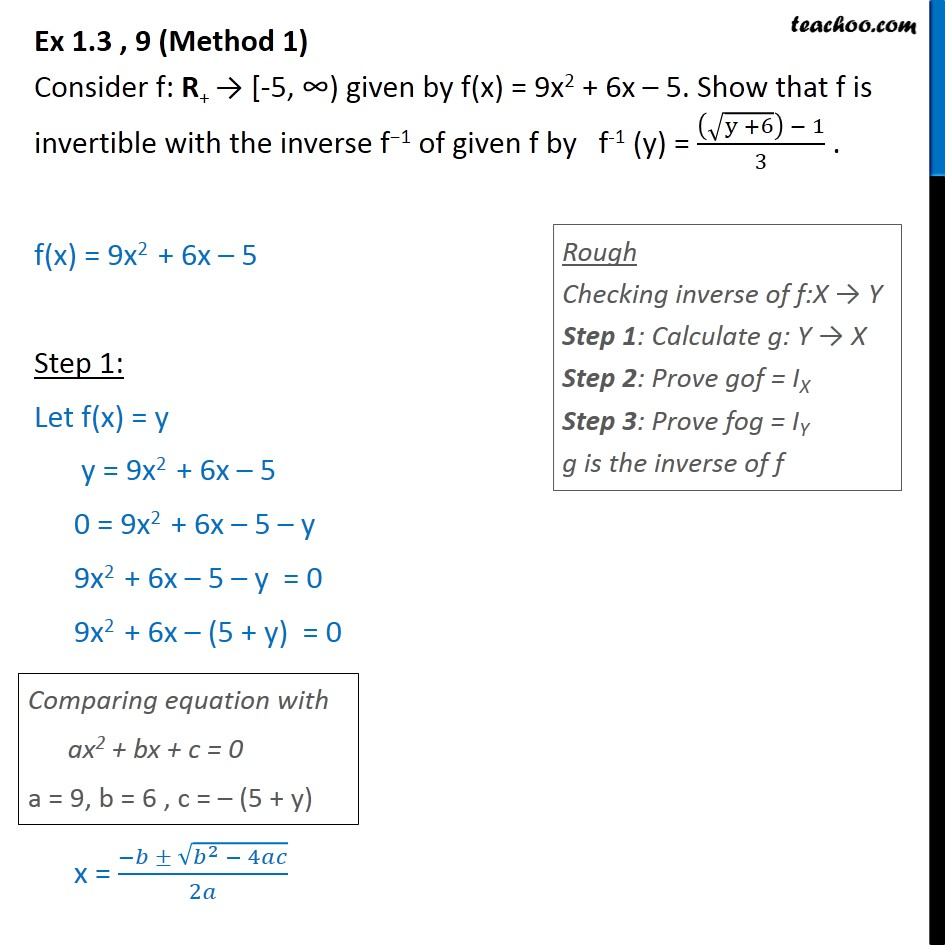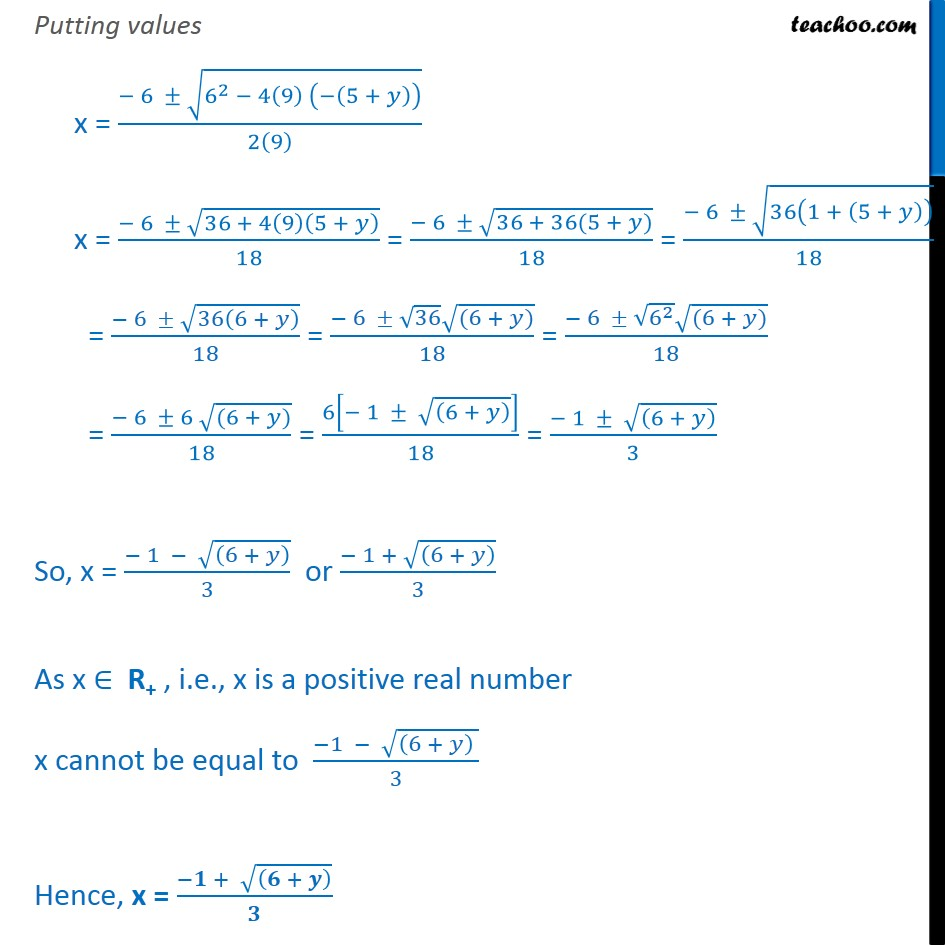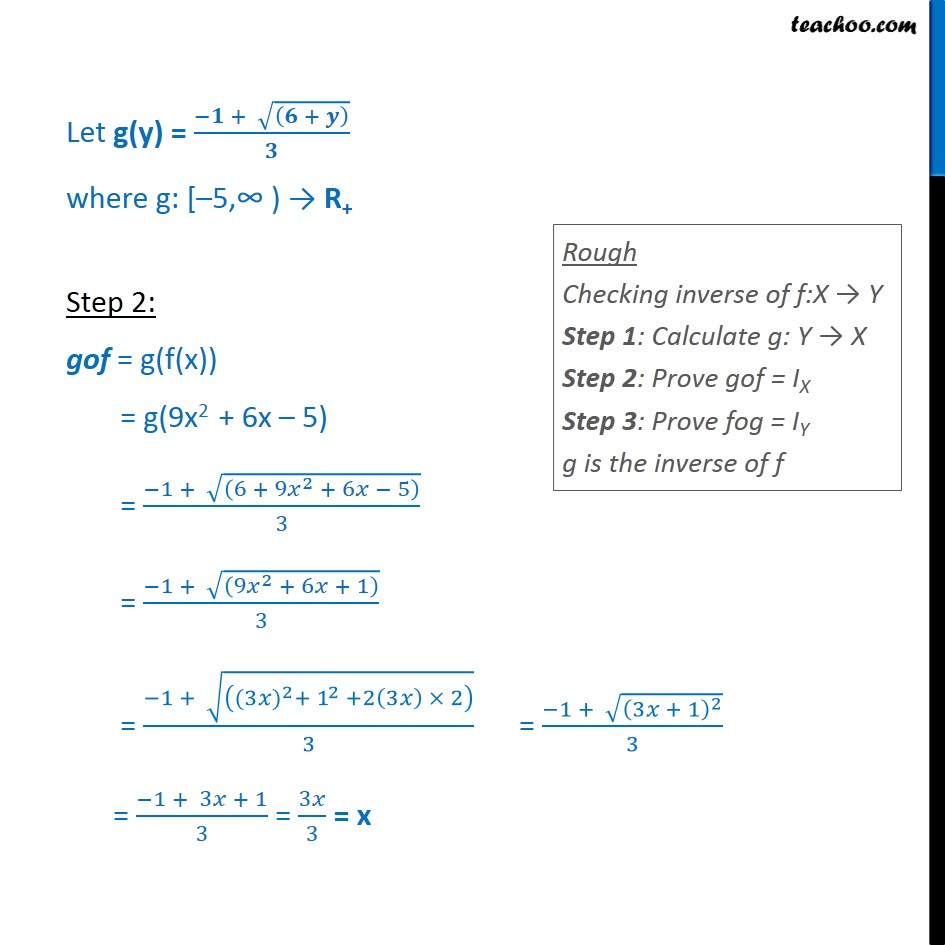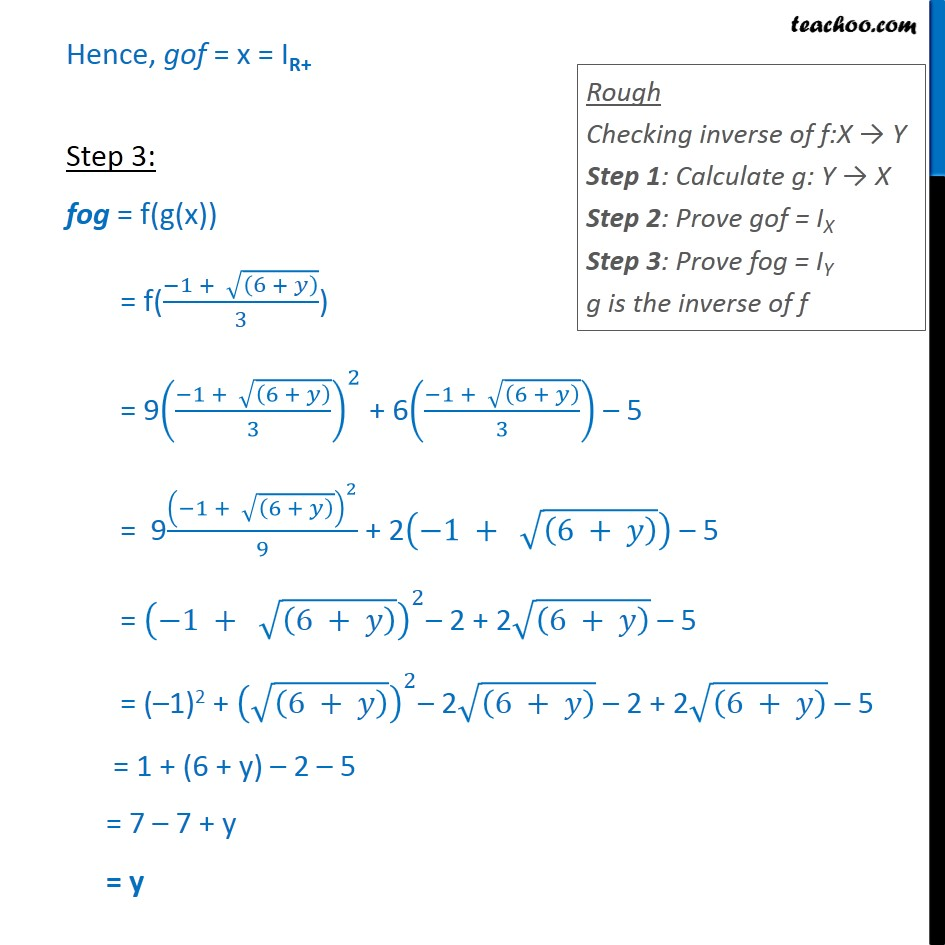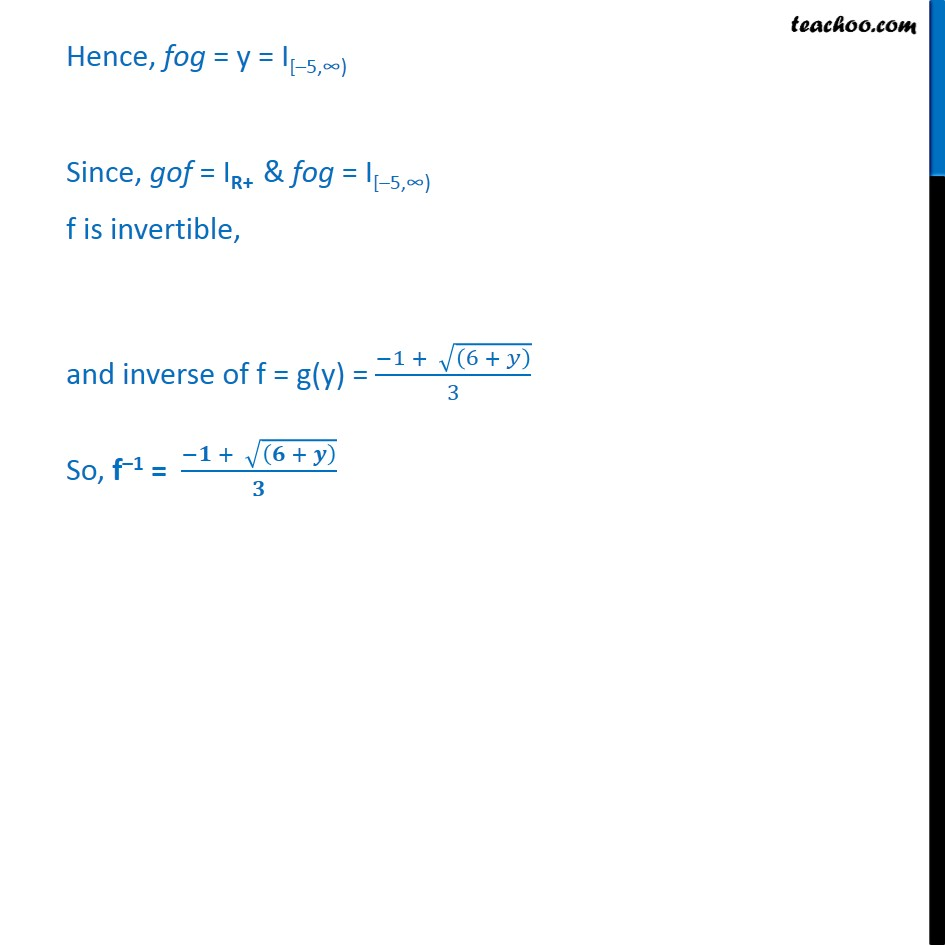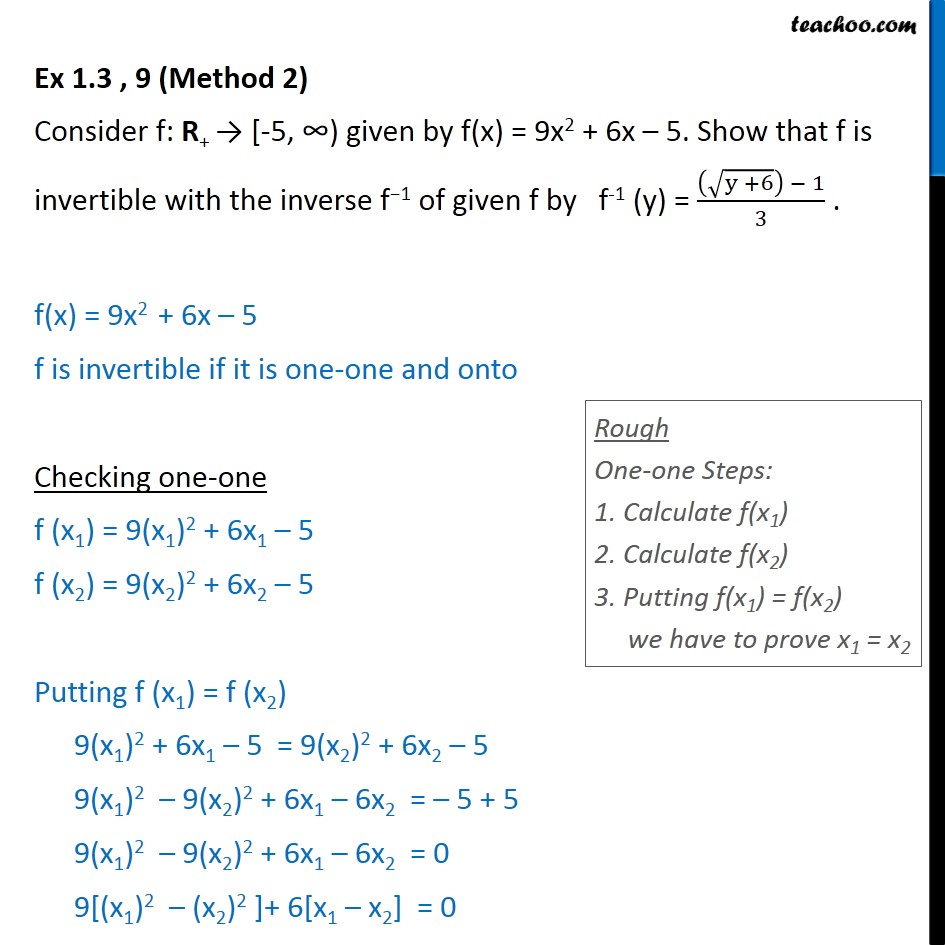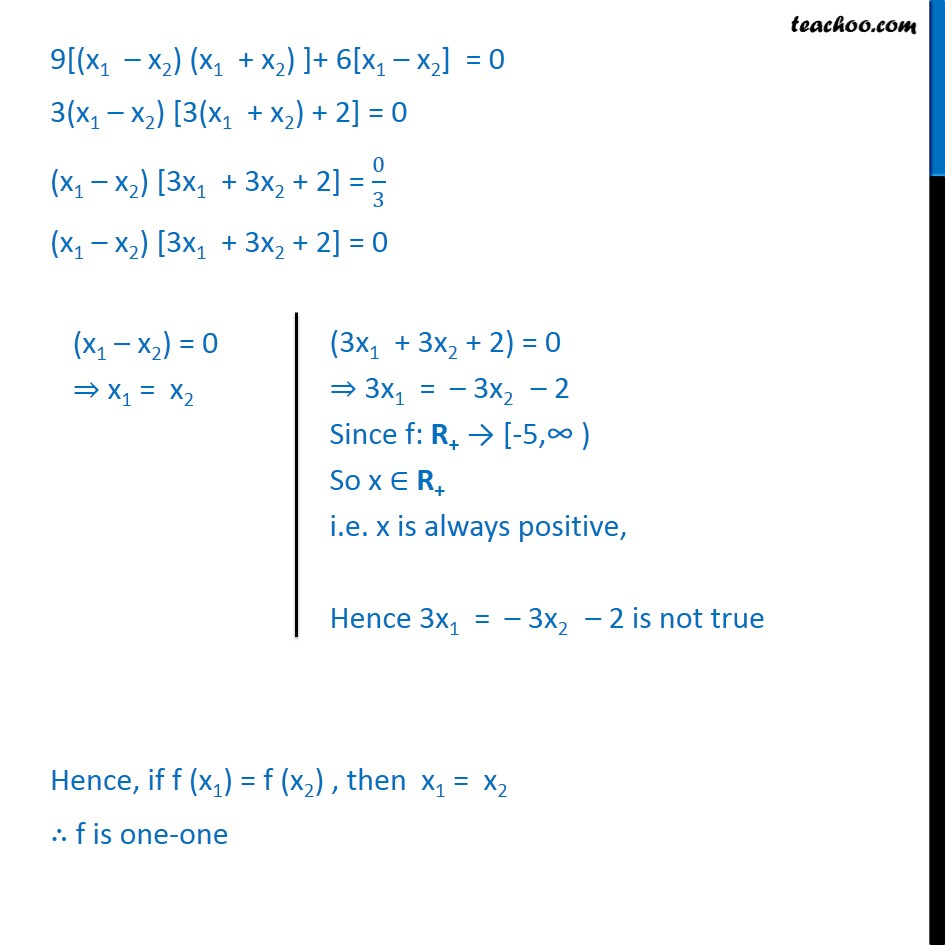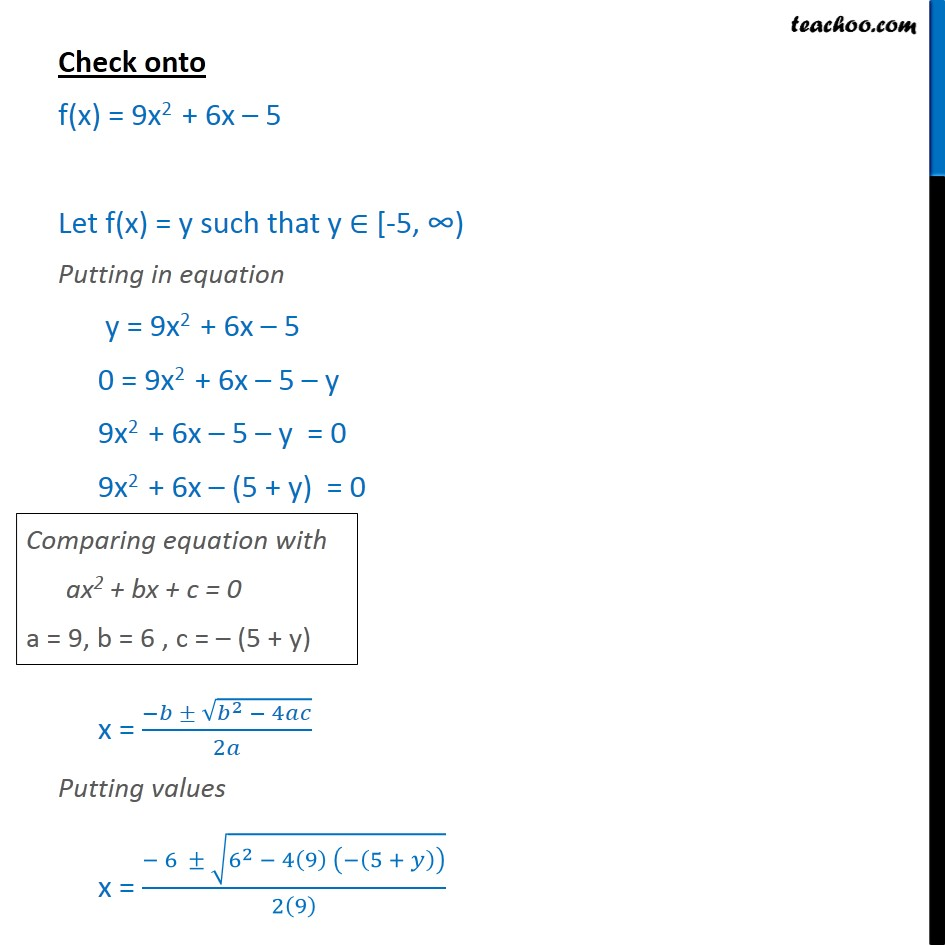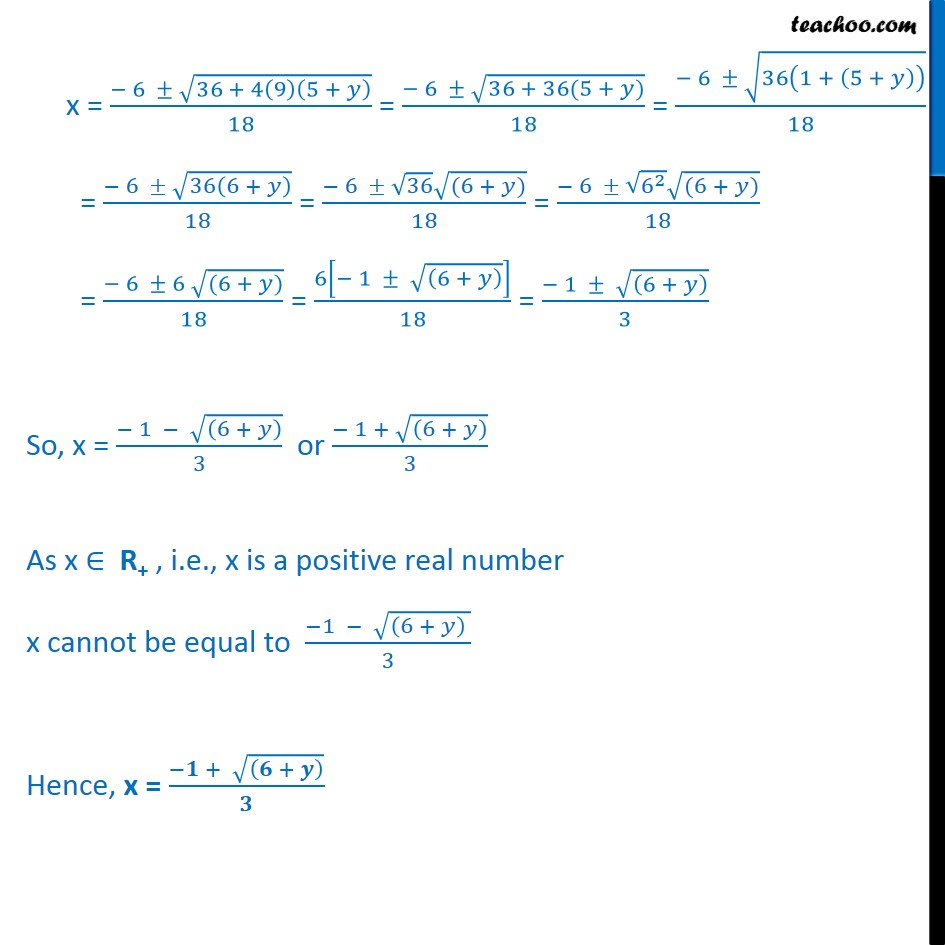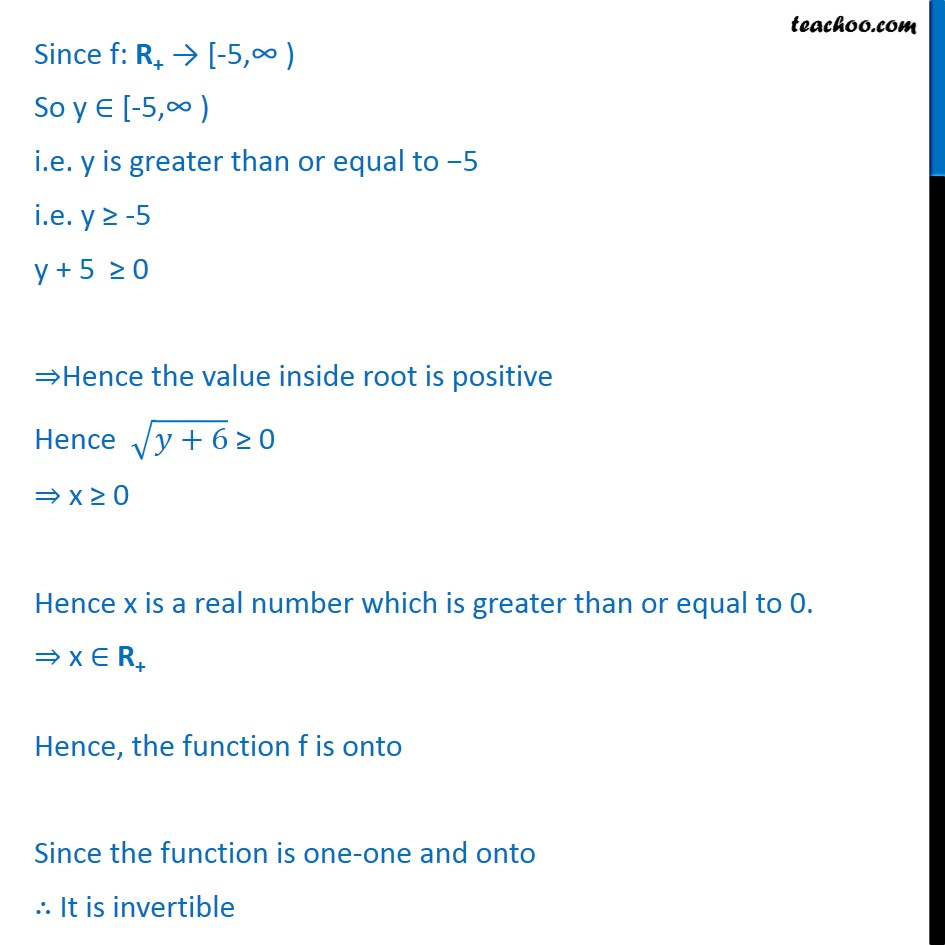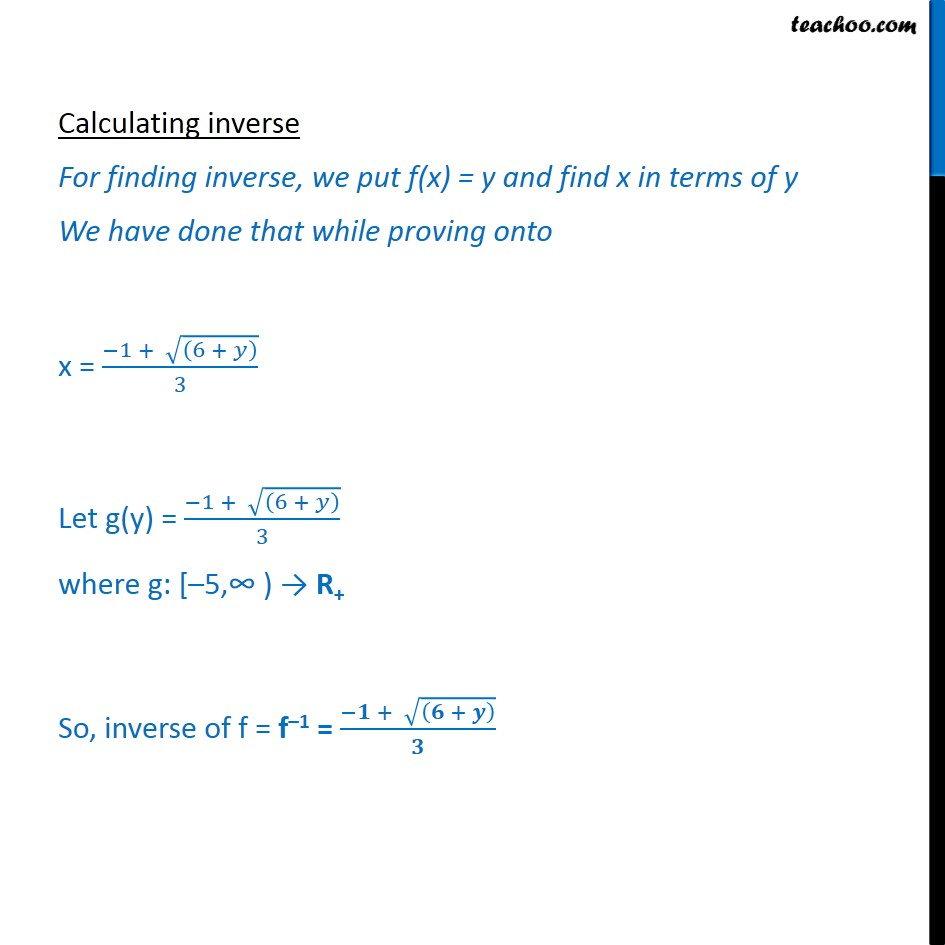1. Chapter 1 Class 12 Relation and Functions
2. Serial order wise
3. Ex 1.3

Transcript

Ex 1.3 , 9 (Method 1) Consider f: R+ [-5, given by f(x) = 9x2 + 6x 5. Show that f is invertible with the inverse f 1 of given f by f-1 (y) = y +6 1 3 . f(x) = 9x2 + 6x 5 Step 1: Let f(x) = y y = 9x2 + 6x 5 0 = 9x2 + 6x 5 y 9x2 + 6x 5 y = 0 9x2 + 6x (5 + y) = 0 x = 2 4 2 Putting values x = 6 6 2 4 9 5 + 2 9 x = 6 36 + 4 9 5 + 18 = 6 36 + 36(5 + ) 18 = 6 36 1 + 5 + 18 = 6 36 6 + 18 = 6 36 (6 + ) 18 = 6 6 2 (6 + ) 18 = 6 6 (6 + ) 18 = 6 1 6 + 18 = 1 6 + 3 So, x = 1 6 + 3 or 1 + 6 + 3 As x R+ , i.e., x is a positive real number x cannot be equal to 1 6 + 3 Hence, x = + + Let g(y) = + + where g: [ 5, R+ Step 2: gof = g(f(x)) = g(9x2 + 6x 5) = 1 + 6 + 9 2 + 6 5 3 = 1 + 9 2 + 6 + 1 3 = 1 + (3 ) 2 + 1 2 +2 3 2 3 = 1 + 3 + 1 2 3 = 1 + 3 + 1 3 = 3 3 = x Hence, gof = x = IR+ Step 3: fog = f(g(x)) = f( 1 + 6 + 3 ) = 9 1 + 6 + 3 2 + 6 1 + 6 + 3 5 = 9 1 + 6 + 2 9 + 2 1 + 6 + 5 = 1 + 6 + 2 2 + 2 6 + 5 = ( 1)2 + 6 + 2 2 6 + 2 + 2 6 + 5 = 1 + (6 + y) 2 5 = 7 7 + y = y Hence, fog = y = I[ 5, Since, gof = IR+ & fog = I[ 5, f is invertible, and inverse of f = g(y) = 1 + 6 + 3 So, f 1 = + + Ex 1.3 , 9 (Method 2) Consider f: R+ [-5, given by f(x) = 9x2 + 6x 5. Show that f is invertible with the inverse f 1 of given f by f-1 (y) = y +6 1 3 . f(x) = 9x2 + 6x 5 f is invertible if it is one-one and onto Checking one-one f (x1) = 9(x1)2 + 6x1 5 f (x2) = 9(x2)2 + 6x2 5 Putting f (x1) = f (x2) 9(x1)2 + 6x1 5 = 9(x2)2 + 6x2 5 9(x1)2 9(x2)2 + 6x1 6x2 = 5 + 5 9(x1)2 9(x2)2 + 6x1 6x2 = 0 9[(x1)2 (x2)2 ]+ 6[x1 x2] = 0 9[(x1 x2) (x1 + x2) ]+ 6[x1 x2] = 0 3(x1 x2) [3(x1 + x2) + 2] = 0 (x1 x2) [3x1 + 3x2 + 2] = 0 3 (x1 x2) [3x1 + 3x2 + 2] = 0 Hence, if f (x1) = f (x2) , then x1 = x2 f is one-one Check onto f(x) = 9x2 + 6x 5 Let f(x) = y such that y [-5, Putting in equation y = 9x2 + 6x 5 0 = 9x2 + 6x 5 y 9x2 + 6x 5 y = 0 9x2 + 6x (5 + y) = 0 x = 2 4 2 Putting values x = 6 6 2 4 9 5 + 2 9 x = 6 36 + 4 9 5 + 18 = 6 36 + 36(5 + ) 18 = 6 36 1 + 5 + 18 = 6 36 6 + 18 = 6 36 (6 + ) 18 = 6 6 2 (6 + ) 18 = 6 6 (6 + ) 18 = 6 1 6 + 18 = 1 6 + 3 So, x = 1 6 + 3 or 1 + 6 + 3 As x R+ , i.e., x is a positive real number x cannot be equal to 1 6 + 3 Hence, x = + + Since f: R+ [-5, So y [-5, i.e. y is greater than or equal to 5 i.e. y -5 y + 5 0 Hence the value inside root is positive Hence +6 0 x 0 Hence x is a real number which is greater than or equal to 0. x R+ Hence, the function f is onto Since the function is one-one and onto It is invertible Calculating inverse For finding inverse, we put f(x) = y and find x in terms of y We have done that while proving onto x = 1 + 6 + 3 Let g(y) = 1 + 6 + 3 where g: [ 5, R+ So, inverse of f = f 1 = + +

Ex 1.3